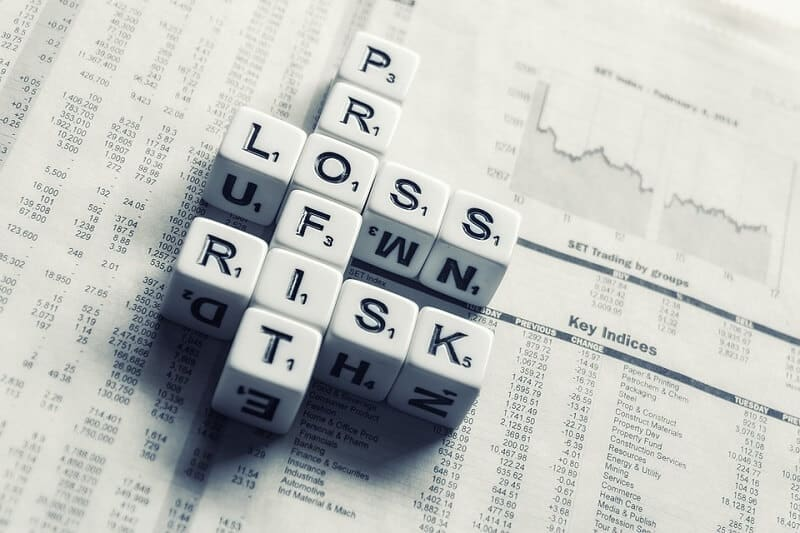## The percentage of profit or loss is always calculated on cost priceIt is always useful to discover the percentage rise or drop. That is called profit and loss.
To calculate profit and loss we have to make clear some terms involved in the calculation.

We will use the stock as an example.

* Cost Price ( CP): The price at which you buy a stock is the cost price. That is the amount paid for purchasing stock.

* Selling price (SP): The Price at which you sell a stock is the sales price. That is the amount received when a stock is sold.

* Profit (also the gain): You get a profit when you sell a stock at a price higher than its cost price. You will like to sell your stock at a higher price. CP < SP

* Loss: If you sell a stock at a price lower than buying price, then you caught a loss. CP > SP

### The percentage of profit or loss is always calculated on the cost price.

The formula for profit is

#### Profit  = SP – CP

The formula for loss is

#### Loss =  CP – SP

Let’s calculate the percentage of loss and the percentage profit.  Percentage Loss and Percentage Profit are calculated based on CP

#### Loss% = (Loss/CP) × 100

For example, one trader purchased a share of stocks for \$1.000 and then sold it or \$1.250.

What is the profit and profit in percentages? Is it 3%, 15%, 18%, 20%, 25%?

OK, this is basic.

In our case, CP is \$1,000.

The trader sold the stock at \$1.250.

The word “sell” is indicated as SP, selling price.

In our case, SP is \$1.250.

We can easily find the profit. It is SP – CP, so

profit = \$1.250 – \$1.000 = \$250

Don’t miss this What Is APY and How to Calculate it

### Now, we have to find the profit percentage.

The formula is

#### [(profit)/CPx100]

so

[(250/1000)x100] = 25%

But what would happen if our trader sold the stock at \$800?

CP is \$1.000

SP is \$800

#### loss = CP – SP

loss = \$1000 – \$800 = \$200

or

[(200/1000)x100] = 20%

### Calculate the Loss and Profit in Percentages

• Divide the amount that you have profited on the investment by the amount invested. To calculate the profit, subtract from the price for which you sold the price that you initially paid for it.
• Now that you have your profit, divide the profit by the initial amount of the investment.
• The last step, multiply the number you got by 100 to see the percentage difference in the investment.